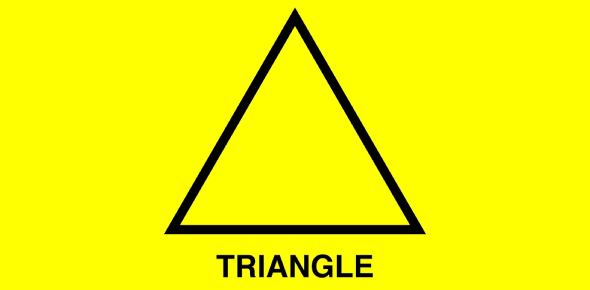# Triangle Quiz

13 Questions | Total Attempts: 4057SettingsTake this test to see how much you know about triangles. (recommended for elementary school students)

• 1.
If you add up the degrees of each angle on a triangle, what is the sum?
• A.

90'

• B.

180'

• C.

360'

• 2.
What are the three types of triangles?
• A.

Equilatiral, isoseles. scalene

• B.

Equalateral, isoscoles, scalene

• C.

Equilateral, isosceles, scalene

• 3.
True or false: all triangles have three sides and three angles.
• A.

True

• B.

False

• 4.
If the three sides of a triangle are all different lengths, they always form an equilateral, isosceles, or scalene triangle.
• A.

True

• B.

False

• 5.
What triangle is it called when all three sides are the same?
• A.

Scalene

• B.

Diagron

• C.

Isosceles

• D.

Equilateral

• 6.
What triangle is it called when two sides are the same and only one is different?
• A.

Scalene

• B.

Diagron

• C.

Isosceles

• D.

Equilateral

• 7.
List the angle names (obese, acute, right) from smallest to biggest in terms of the angle type.
• A.

Obese, acute, right

• B.

Acute, obese, right

• C.

Right, obese, acute

• D.

Acute, right, obese

• E.

Obese, right, acute

• F.

Right, acute, obese

• 8.
On a triangle, there are angles. There are different types of angles (acute, obese, right). Is the acute angle bigger or smaller than the right angle?
• A.

Smaller

• B.

Bigger

• 9.
On a triangle, there are angles, there are different kinds of angles (acute, right, obese). What is the degrees of the right angle?
• A.

A right angle is 80'.

• B.

A right angle is 90'

• C.

A right angle is 100'

• D.

A right angle is 110'

• 10.
• 11.
There are different types of pyramids (3D triangles). Which ones are actually types of pyramids?
• A.

Triangle pyramid

• B.

Square pyramid

• C.

Circular pyramid

• D.

Triangular pyramid

• 12.
What is a pyramid with a circular bottom called?
• A.

A circular pyramid

• B.

A cone pyramid

• C.

A cone

• D.

A circle pyramid

• 13.
Mark everything that is correct about triangles.
• A.

They have three sides

• B.

An acute angle on a triangle can be up to 100' before it becomes a right angle.

• C.

Every angle on a triangle has to be at least 10'

• D.

A right angle on a triangle is 90', so the biggest an acute angle on a triangle can be is 90'

• E.

They are ALWAYS either equilateral, isosceles, or scalene triangles.

• F.

Every angle on a triangle has to be less then 100'

• G.

Triangles don't have to have three angles

• H.

Every angle on a triangle has to be less than 180'

• I.

The smallest an obese angle on a triangle can be is 80' degrees because a right angle is 80'.

• J.

They have three angles

• K.

All triangles have three sides except for a diagron triangle, which has three and a half sides.

Related Topics